In this Review, we review some recent rigorous results on large N problems in quantum field theory, stochastic quantization, and singular stochastic partial differential equations (SPDEs) and their mean field limit problems. In particular, we discuss the O(N) linear sigma model on a two- and three-dimensional torus. The stochastic quantization procedure leads to a coupled system of N interacting Φ4 equations. In d = 2, we show uniformity in N bounds for the dynamics and convergence to a mean-field singular SPDE. For large enough mass or small enough coupling, the invariant measures [i.e., the O(N) linear sigma model] converge to the massive Gaussian free field, the unique invariant measure of the mean-field dynamics, in a Wasserstein distance. We also obtain tightness for certain O(N) invariant observables as random fields in suitable Besov spaces as N, along with exact descriptions of the limiting correlations. In d = 3, the estimates become more involved since the equation is more singular. We discuss in this case how to prove convergence to the massive Gaussian free field. The proofs of these results build on the recent progress of singular SPDE theory and combine many new techniques, such as uniformity in N estimates and dynamical mean field theory. These are based on joint papers with Scott Smith, Rongchan Zhu, and Xiangchan Zhu.

In quantum field theory (QFT), there are important models where the “field” takes values in an N-dimensional space. A natural question is that what is the asymptotic behavior of such models as the dimensionality of the target space N. The perspective of investigating these questions in this Review is called stochastic quantization. Stochastic quantization is a procedure that formulates a quantum field theory in terms of a stochastic partial differential equations (SPDEs). More precisely, for a quantum field theory where the target space has dimension N, its stochastic quantization yields a system of N coupled SPDEs. This procedure, then, brings in many powerful analytical tools, including the recent developments of SPDE solution theories and their a priori estimates, and mean field theory techniques. The purpose of this Review is to survey the recent results along this direction.

For most of this Review, we will focus on the linear σ-model in two and three dimensions. However, we will first briefly review the origin of the large N problems in physics.

Large N methods (or “1/N expansions”) in theoretical physics are ubiquitous and are generally applied to models where the dimensionality of the target space is large. It was first noted by Stanley1 for lattice spin models (rather than QFT) that they exhibit considerable simplification as N (the number of spin components) becomes large: at least at the level of free energy, as N, these models become the “spherical model,” which is a solvable model introduced by Berlin and Kac in 1952.2 See Baxter’s book,3 Sec. 5, for an expository discussion on spherical model. Later, the large N convergence to the spherical model was established at the level of free energies4 and, then, of correlations.5,6 Again, in the context of spin systems, the authors of Ref. 7 found a systematic way to expand in powers of 1/N and obtained 1/N order corrections to the spherical model.

In 1973, Wilson exploited this idea in quantum field theory.8 In particular, Wilson studied both bosonic (linear σ-model) and fermionic models. The linear σ-model is the N-component generalization of the $Φd4$ model, given by the (formal) measure

$dνN(Φ) =def 1ZNexp−∫Td∑j=1N|∇Φj|2+m∑j=1NΦj2+λ2N∑j=1NΦj22dxDΦ$
(1.1)

over $RN$ valued fields Φ = (Φ1, Φ2, …, ΦN), where ZN is a normalization constant and $DΦ$ is the formal “Lebesgue measure.” We will discuss below the physics predictions and how they are related with our rigorous results. Soon later, Coleman, Jackiw, and Politzer9 calculated the effective potential and studied spontaneous symmetry breaking of this model for d ⩽ 4; see also Ref. 10 for more extensive analysis in d = 4.11

Around the same time, Gross and Neveu12 studied the 1/N expansion for a two-dimensional fermionic model, where N Dirac fermions interact via a quartic term (“four-fermion interactions”): namely, the Lagrangian is given by $∑i=1Nψ̄i(i∂/)ψi+λN(∑i=1Nψ̄iψi)2$. This model had also been touched in Wilson’s paper.8 It was observed that the model has asymptotic freedom and symmetry breaking (for a discrete chiral symmetry) and produces a fermion mass.

Another class of model considered from the 1970s is the nonlinear σ-models in two dimensions. For instance, see Ref. 13, which considered models where Φ takes values in a sphere in $RN$. It can be viewed as the limit of $RN$ valued σ-model with a potential $K(Φ⋅Φ−a2)2$ in a formal limit K. The idea was also extended to CPN−1-type nonlinear σ-models14,15 (where more general homogeneous space G/H valued models were also formulated).

Matrix-valued field theories are considered to be more difficult. In 1974, t’Hooft16 gave the solution of the two-dimensional Yang–Mills model with the SU(N) gauge group as N. After this seminal work by t’Hooft, the large N behavior of gauge theories was extensively studied by physicists; see, e.g., Refs. 1720. See also Ref. 21 (and the references therein) for a broad class of matrix models.

It is certainly impossible to list all the vast literature; instead, we refer to Ref. 22 for an edited comprehensive collection of articles on large N as applied to a wide spectrum of problems in quantum field theory and statistical mechanics; see also the review articles23 and Ref. 24 or Coleman’s excellent book (Ref. 25, Chap. 8) for summaries of the progress.

A standard approach in quantum field theory is perturbation theory, namely, calculating physical quantities of a given model by perturbative expansion in a coupling constant of the model. In large N problems, terms in perturbation theory can have different orders in 1/N, and the “1/N expansion” is a re-organization of the series in the parameter 1/N, with each term typically being a (formal) sum of infinitely many orders of the ordinary perturbation theory.

To explain this formalism a bit more, we first recall the usual perturbation theory for the Φ4 model with N = 1 and m = 0 (for simplicity) in (1.1). This could be found in a standard QFT textbook, e.g., Peskin and Schroeder.26 Loosely speaking, the perturbative calculation of, for instance, a two-point correlation of Φ is given by a sum of Feynman graphs with two external legs and degree-4 internal vertices that represents the quartic interaction Φ4,

For the vector model (1.1) with N > 1, which was studied by Wilson,8 the perturbative calculation of the two-point correlation of Φi (with i fixed) is also given by the sum of Feynman graphs with two external legs and degree-4 internal vertices, but now, each vertex carrying two distinct summation variables and a factor 1/N that represents the interaction $1N∑j,kΦj2Φk2$ term in (1.1),

Heuristically, graph (a) is of order $1N∑j≈O(1)$ and graph (b) is of order $1N2∑j≈O(1N)$. The philosophy of Ref. 8 is that graphs with “self-loops” such as (a) get canceled by Wick renormalization,27 and all other graphs with internal vertices such as (b) are at least of order O(1/N) and, thus, vanish, so the theory would be asymptotically Gaussian free field. (We rigorously prove this in Secs. VI and VIII C in 2D and 3D.)

Since model (1.1) is invariant under any O(N) rotation of Φ, it is more natural to study the O(N)-invariant observables than the field itself, such as $∑j=1NΦj2$. One needs to find a suitable scaling for such observables to see interesting fluctuations. In the “law of large numbers behavior” scaling $1N∑j=1NΦj2$, one would expect a deterministic limit. Interesting fluctuation, then, arises in the next scale.

Following the above heuristic, we assume that the model is Wick renormalized, and so the perturbation series does not contain self-loops, and we consider $1N∑j=1N:Φj2:$. Note that here $:Φj2:$ is the Wick square. The bubbled diagrams of the following form are all the O(1) contributions to its two-point correlation:

In fact, it is known in physics literature that these “bubble chains” are dominant (after Wick renormalization) contributions for this model: for instance, if we consider another observable $1N:∑i=1NΦi22:$, the leading contribution to its one-point correlation would, then, be the “bubble loops,” i.e., the above type of diagrams with x, y identified.

Besides directly examining the perturbation theory, alternative (and more systematic) methodologies of analyzing such expansion were discovered in physics. We briefly recall some of them.

In Ref. 28, Schwinger–Dyson equations were used to study models of the type (1.1). These are essentially a type of integration by parts formulas. They yield a hierarchy of relations for correlation functions of different orders. This hierarchy does not close, so it is somewhat similar to perturbation theory, but one advantage of using Schwinger–Dyson equations is that one could again write a truncation remainder in terms of correlations of the field. This will be useful for rigorous studies (see Ref. 29), and we will come back to this point in Sec. VII.

Schwinger–Dyson equations are particularly powerful when we move from vector valued models of the type (1.1) to matrix valued models, such as Yang–Mills model. In the context of Yang–Mills model, the authors of Ref. 19 discovered relations between Wilson loop observables among different loops, now called Makeenko–Migdal equations or master loop equations. These are also broadly used in the study of random matrices, for instance, see the book30 by Guionnet.

The problem of the perturbative argument discussed in Sec. I B (besides being non-rigorous) is that keeping track of all the diagrams and proving that there is no other diagrams appearing at the leading order are a combinatoric challenge; this challenge also exists when iteratively applying Schwinger–Dyson equations. A better way is to introduce a “dual” field or “auxiliary” field, which we briefly review now. A very nice exposition of this idea can be found in Coleman’s book (Ref. 25, Chap. 8) [see also the original paper9 or the review (Ref. 24, Sec. 2)]. The exact ways of implementing this idea vary among the literature, but roughly speaking, one will shift the action in (1.1) by a quadratic term involving a new field σ when calculating an expectation with respect to the measure N,

$∫(⋯)dνN(Φ)→∫exp−∫N2λ(σ−λN∑j=1NΦj2)2dσdνN(Φ).$

This is because the integral over σ is simply a Gaussian integral, which yields a constant that can be absorbed into the partition function. We, then, obtain $exp(−S)$, where

$S(σ,Φ)=∫∑j=1N|∇Φj|2+m∑j=1NΦj2+N2λσ2−12∑j=1NσΦj2dx.$

In particular, the quartic term is canceled. Still considering two-point correlation of Φi, for instance, one has the following type of diagrams:

Here, the dashed line represents the covariance of the “Gaussian measure” $exp(−∫N2λσ(x)2dx)$, which is simply $λNδ$. Each internal vertex is degree 3 because of the $σΦj2$ interaction term.

One could study this theory of (σ, Φ), but as explained in Ref. 25, since the above action is quadratic in Φ, one could “integrate out” (Φ1, …, ΦN) and obtain an “effective action” for σ. This Gaussian integral would, however, yield a non-local interaction because of the term |∇Φj|2, namely, a determinant $det(−Δ+m−σ2)N/2$ or $det(1−12(−Δ+m)−1σ)N/2$ if we factor out the determinant of the massive Laplacian. Many physical properties can be deduced from this dual field theory for σ, for instance, one could exponentiate this determinant to obtain a measure of the form eNF(σ), where F has the form tr log(⋯) so that large N limit becomes a question of critical point analysis for F. The 1/N expansion for correlations will also turn out to be a simple perturbative expansion in the dual picture.

A rigorous study of large N in mathematical physics was initiated by Kupiainen29,31 around 1980. See also his review.32 The literature mostly related to the present article is Ref. 29, which studied the linear sigma model in continuum in d = 2 (with Wick renormalization) and proved that the 1/N expansion of the pressure (i.e., vacuum energy or log of partition per area) is asymptotic, and each order in this expansion can be described by sums of infinitely many Feynman diagrams of certain types. This paper uses both Dyson–Schwinger equations and the dual field method, together with constructive field theory methods, such as chessboard estimates33 based on reflection positivity. Borel summability of the 1/N expansion of Schwinger functions for this model was discussed in Ref. 34.

Most of the other rigorous results are proved on lattices and, thus, ignore the small scale singularity (which is one of the key difficulties in our work). We also review some of these results here even if they are not necessarily directly related to our results. In Ref. 31, Kupiainen considered the N-component nonlinear sigma model (fields take values in a sphere in $RN$) on the d-dimensional lattice with fixed lattice spacing. As mentioned above, this simplifies to “spherical model” as N, and for the spherical model, it is known that the critical temperature is 0 when d ⩽ 2 and is strictly positive when d > 2. The author of Ref. 31 proved that the large N expansion of correlation functions and free energy is asymptotic above the spherical model critical temperature (meaning all temperature if d ⩽ 2), and a mass gap was established for these temperatures when N is sufficiently large. The main idea therein was the dual field representation (for which the heuristic is discussed in Sec. I C), which yields nonlocal expectations, and this technical difficulty was solved using random walk expansion ideas from Brydges and Federbush.35 Asymptoticity was later extended to Borel summability by Ref. 36.

In the 1990s, Kopper, Magnen, and Rivasseau37 proved the mass gap for fermions in the Gross–Neveu model when N is sufficiently large, and the model has at least two pure phases. They again work with the dual field as illustrated above (which ends up with a determinant of Dirac operator) with suitable ultraviolet regularization and apply cluster and Mayer expansion techniques. Note that the Gross–Neveu model action has a (discrete) chiral symmetry, which prohibits a mass, but the mass is acquired in a symmetry breaking. The authors of Ref. 38 (on lattice Z2)39 [on $R2$ but with a certain ultraviolet cutoff and a “soft constraint” K(Φ · Φ − 1)2 in the potential], then, revisited the nonlinear σ model and showed a mass gap for N sufficiently large, and they both work with dual fields and determinants using cluster expansions.

A large N limit and expansion for the Yang–Mills model have drawn much attention, following t’Hooft’s 1974 work.16 In two space dimensions (continuum plane), Lévy40 proved the convergence of Wilson loop observables to the master field in the large N limit, with orthogonal, unitary, and symplectic structure groups. The master field is a deterministic object, which was described conjecturally by Singer in 199541 who pointed out its connections with free probability. Lévy’s work40 also rigorously establishes integration by parts, i.e., Schwinger–Dyson equations both for finite N and in the large N limit for the expectations of Wilson loops, which in this context are called the Makeenko–Migdal equations. Note that the two-dimensional Yang–Mills model has special integrability structures, which allows one to make sense of the random Wilson loop observables in continuum—this was established by Lévy in Refs. 42 and 43, which Ref. 40 relies on. Around the same time, Anshelevich and Sengupta44 also gave a construction of the master field in the large N limit via a different approach, which is based on the use of free white noise and of free stochastic calculus; these methods were developed earlier by Sengupta in Refs. 45 and 46. On the lattice of any dimension, we refer to Ref. 47 (respectively, Ref. 48) for computation of correlations of the Wilson loops in the large N limit (respectively, 1/N expansion), which relates to string theory.

We remark that while the Schwinger–Dyson equations for vector models (as we will discuss more in Sec. VII) are easy to derive, Makeenko–Migdal equations for Yang–Mills models are more difficult to derive (rigorously). The first rigorous version was established for the two-dimensional Yang–Mills model in continuum in Ref. 40, and alternative proofs and extensions were given in Refs. 4952 on plane or surfaces. On lattice, for finite N and in any dimension, these were derived by Chatterjee (Refs. 47 and 53) and, then, in Ref. 54 using stochastic ordinary differential equations (ODEs) on Lie groups.

Stochastic quantization refers a procedure to turn Euclidean quantum field theories to singular SPDEs and, thus, bring stochastic analysis and PDE methods into the study. They were introduced by Parisi and Wu in Ref. 55. Given an action $S(Φ)$, which is a functional of Φ, one considers a gradient flow of $S(Φ)$ perturbed by space-time white noise ξ,

$∂tΦ=−δS(Φ)δΦ+ξ.$
(2.1)

Here, $δS(Φ)δΦ$ is the variational derivative of the functional $S(Φ)$; for instance, when $S(Φ)=12∫(∇Φ)2dx$ is the Dirichlet form, $δS(Φ)δΦ=−ΔΦ$ and (2.1) boils down to the stochastic heat equation,

$∂tΦ=ΔΦ+ξ.$

Note that Φ can also be multi-component fields, with ξ being the likewise multi-component. Another example is the “dynamical Φ4 equation,”

$∂tΦ=ΔΦ−λΦ3+ξ,$
(2.2)

and it arises from the gradient of $∫12|∇Φ|2+λ4Φ4dx$, which is model (1.1) with m = 0, N = 1.

The significance of these “stochastic quantization equations” (2.1) is that given an action $S(Φ)$, the formal measure that defines an Euclidean quantum field theory

$1Ze−S(Φ)DΦ$
(2.3)

is formally an invariant measure56 for Eq. (2.1). Here, DΦ is the formal Lebesgue measure and Z is a “normalization constant.” We emphasize that (2.3) are only formal measures because, among several other reasons, there is no “Lebesgue measure” DΦ on an infinite-dimensional space, and it is a priori not clear at all if the measure can be normalized. The task of constructive quantum field theory is to give precise meaning or constructions to these formal measures. This area of study has yielded numerous deep results, which we do not mean to give a full list of literature; we only refer to the book.57,58

Regarding the well-posedness of (2.2), in two dimensions, two classical works are Ref. 59 where martingale solutions are constructed and Ref. 60 where local strong solutions are obtained, as well as the more recent approach to global well-posedness in Ref. 61. In three dimension, the local strong solution is obtained as an application of regularity structures62 and, then,63 as an application of paracontrolled analysis.

Given the very recent progress of SPDE, a new approach to construct the measure of the form (2.3) is to construct the long-time solution to the stochastic PDE (2.1) and average the distribution of the solution over time. This approach has shown to be successful for the Φ4 model in d ⩽ 3 in a series of very recent studies, which starts with Ref. 64 on the 3D torus where a priori estimates were obtained to rule out the possibility of finite time blow-up. Then, in Refs. 65 and 66, the authors established a priori estimates for solutions on the full space $R3$, yielding the construction of Φ4 quantum field theory on the whole $R3$ and verification of some key properties that this invariant measure must satisfy as desired by physicists, such as reflection positivity. See also Ref. 67. Similar uniform a priori estimates are obtained by Ref. 68 using the maximum principle.

Large N problems in the stochastic quantization formalism have also been discussed (on a heuristic level) in the physics literature, for instance, Refs. 69 and 70 and Ref. 71, Sec. 8. The review on large N by Moshe and Zinn-Justin (Ref. 24, Sec. 5.1) is close to our setting; it makes an “ansatz” that $1N∑j=1NΦj2$ in the equation would self-average in the large N limit to a constant; our results justified this ansatz and in the non-equilibrium setting generalize it.

As we will see, the methods in the proofs of our main theorems borrow some ingredients from mean field limit theory (MFT). To the best of our knowledge, the study of mean field problems originated from the work of McKean.72 Typically, a mean field problem is concerned with a system of N particles interacting with each other, which is often modeled by a system of stochastic ordinary differential equations, for instance, driven by independent Brownian motions. A prototype of such systems has the form $dXi=1N∑jf(Xi,Xj)dt+dBi$; see, for instance, the classical reference by Sznitman [Ref. 73, Sec. I(1)], and in the N limit, one could obtain decoupled SDEs each interacting with the law of itself: we will briefly review this calculation below.

In simple situations, the interaction f is assumed to be “nice,” for instance, globally Lipschitz;72 much of the literature aims to prove such limits under more general assumptions on the interaction; see Ref. 73 for a survey.74

We note that mean field limits are studied under much broader frameworks or scopes of applications, such as the mean field limit in the context of rough paths (e.g., Refs. 7577), mean field games (e.g., survey78), and quantum dynamics (e.g., Ref. 79 and the references therein). We do not intend to have a comprehensive list, but rather refer to survey articles80,81 and the book (Ref. 82, Chap. 8) in addition to Ref. 73.

The study of the mean field limit for SPDE systems also has precursors; see, for instance, the book (Ref. 83, Chap. 9) or Ref. 84. However, these results make strong assumptions on the interactions of the SPDE systems, such as linear growth and globally Lipschitz drift, and certainly do not cover the singular regime where renormalization is required as in our case.

Before we delve into the mean field limit for singular SPDE systems, we briefly review a very simple example regarding SDEs, which is taken from the work of Sznitman [Ref. 73, Sec. I(1)]. We will see that some of the simple ideas will be reflected when we move on to the much more technical SPDE setting. Consider

$dXi=1N∑j=1Nf(Xi,Xj)dt+dBi(i=1,…,N),$

where Bi (i = 1, …, N) are independent Brownian motions. One can show that as N, the solution converges to that of (“McKean–Vlasov limit”)

$dX̄i=∫Rf(X̄i,y)ut(y)dydt+dBi(i=1,…,N),$
(3.1)

where $ut=Law(X̄i(t))$. Note that this is a decoupled system now. To prove the above result, we subtract the two systems and get

$∂t(Xi−X̄i)=1N∑j=1Nf(Xi,Xj)−∫Rf(X̄i,y)ut(y)dy−1N∑j=1Nf(X̄i,Xj)+1N∑j=1Nf(X̄i,Xj)−1N∑j=1Nf(X̄i,X̄j)+1N∑j=1Nf(X̄i,X̄j).$
(3.2)

Here, the terms in the second line are put “by hand” and they obviously sum to zero. We, then, compare the first and third terms on the RHS and the fourth and fifth terms and the second and the last term; we show that the differences all vanish as N. The most important case is as follows:

$E1N∑j=1Nf(X̄i,X̄j)−∫Rf(X̄i,y)ut(y)dy2∼1/N.$
(3.3)

Note that the nontriviality here is that the left-hand side “appears to be” 1/N2 times two summations, which would be O(1) as N, but actually, we have a decay of 1/N. To show this, note that $f(X̄i,X̄j)−∫Rf(X̄i,y)ut(y)dy$ is mean-zero, so for j1j2,

$Ef(X̄i,X̄j1)−∫Rf(X̄i,y)ut(y)dyf(X̄i,X̄j2)−∫Rf(X̄i,y)ut(y)dy=0$
(3.4)

since $X̄j1$ and $X̄j2$ are independent.

To bound the other terms on the RHS of (3.2), we need some assumptions on f, for instance, we assume that f is globally Lipschitz. Then, we can easily bound the other terms on the RHS of (3.2) by $|Xj−X̄j|$. Upon taking expectation and by symmetry of law, these are just $E|Xi−X̄i|$. We could, then, apply Gronwall’s lemma to show that $Esupt∈[0,T]|Xi(t)−X̄i(t)|∼1/N$.

The key to the above argument is the decay in (3.3), which relies on the mechanism demonstrated in (3.4) for L2 products of independent and mean-zero random variables. One also needs some way to “move” other terms from the RHS to the LHS using Gronwall’s lemma or some other methods. We will see that the estimates in singular SPDEs will be much more complicated, but these basic mechanisms will still be present. For instance, our Theorem 5.1 can be viewed as a result of this flavor, in an SPDE setting, and in fact, the starting point of our proof is, indeed, close in spirit to Ref. 73, Sec. I(1) where one subtracts Xi from Yi to cancel the noise and, then, bound a suitable norm of the difference.

Before discussing large N problems, we first briefly review the solution theory for the stochastic quantization of Φ4 on T2, i.e., the case N = 1. For simplicity, here and in Secs.. VVII, we set λ = 1. Equation (2.2) is only formal in d = 2 since Φ is expected to be distributional. To give a meaning to it, one should regularize and insert renormalization terms (also often called “counter-terms” in the context of quantum field theory85). Namely, we take a sequence of mollified noises ξɛξ (for instance, convolving ξ with smooth functions χɛ, which approximate Dirac distribution as ɛ → 0) and consider the mollified equation,

$∂tΦε=ΔΦε−(Φε3−CεΦε)+ξε,$
(4.1)

where Cɛdiverges as ɛ → 0 at a suitable rate, with some initial condition Φɛ(0) = ϕ.86 If the sequence of constants Cɛ is suitably chosen, the sequence of smooth solutions Φɛ of (4.1) will converge to a nontrivial limit Φ = limɛ→0Φɛ.

The following argument is originally given by Da Prato and Debussche.60 Write $L=∂t−Δ$, and let Zɛ be the stationary solution to the mollified linear stochastic heat equation $LZε=ξε$. The key observation is that the most singular part of Φ is Z, so if we decompose

$Φε=Zε+Yε,$
(4.2)

we can expect the remainder Yɛ to converge in a space of better regularity. Substituting (4.2) into (4.1) yields

Here, we have defined

We will choose $Cε=E[Zε2(0,0)]$, and the reason will be clear below. Equation (4.3) now looks more promising since noise ξɛ (whose limit is rough) has dropped out. This manipulation has not solved the problem of multiplying distributions since the limit of Zɛ is still a distribution, which is why we need renormalization when define its powers. However, Zɛ is a rather concrete object since it is Gaussian distributed. This makes it possible to study the renormalization and convergence of $Zε2$ and $Zε3$ via probabilistic methods. For example, consider the expectation

$E[Zε(t,x)2]=∫P(t−s,x−y)P(t−r,x−z)χε(2)(s−r,y−z)dsdydrdz,$
(4.4)

where $χε(2)$ is the convolution of the mollifier χɛ with itself and P is the heat kernel. Due to the singularity of the heat kernel P at the origin, this integral diverges like O(log ɛ) as ɛ → 0 in two spatial dimensions. The constant Cɛ defined above simply subtracts this divergent mean from $Zε2$ and similarly for the other term $Zε3$. This amounts to considering the renormalized Φ4 equation [Eq. (4.1)].

It can be shown that Zɛ,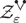, and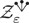do converge to nontrivial limits in probability as space-time distributions of regularity Cκ for any κ > 0.87 In fact, thanks to Gaussianity of Zɛ, given a smooth test function f, one can explicitly compute any probabilistic moment of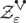(f) and prove its convergence. By choosing f from a suitable set of wavelets or Fourier basis, one can apply a version of Kolmogorov’s theorem to prove that Zɛ,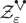, and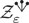converge in Cκ. We denote these limits by Z,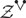, and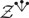.

Passing (4.3) to the limit, we get

We can prove the local well-posedness of this equation as a classical PDE by a standard fixed point argument. For this, we use a classical result in harmonic analysis under the name “Young’s theorem” (e.g., Ref. 62, Proposition 4.14 and Ref. 88, Theorems 2.47 and 2.52), which states that if $f∈Cα$, $g∈Cβ$, and α + β > 0, then $f⋅g∈Cmin(α,β)$. Thus, if we assume that $Y∈Cβ$ for β ∈ (0, 2), then the worst term in the parentheses in (4.5) has regularity −κ. By the classical Schauder estimate, which states that the heat kernel improves regularity by 2, the fixed point map

is well defined on Cβ since Cκ+2Cβ. With a bit of extra effort, one can show that over short time interval, the fixed point map is contractive and, thus, has a fixed point in Cβ, which is the solution. To conclude, one has Φ = Z + Y, which is the local solution to the renormalized Φ4 equation on T2; the above renormalization is well known as “Wick renormalization,” and a commonly used notation is to write the equation as

$∂tΦ=ΔΦ−:Φ3:+ξ.$
(4.7)

The global (long-time) bound is first established by Mourrat and Weber.61 The start point is a PDE energy estimate—here, it will be an Lp estimate for Y. Namely, we multiply (4.5) by Yp−1 and integrate over space,

where ⟨, ⟩ denotes the L2 product. Note that the second term on the LHS arises from ΔY and the third term on the LHS arises from −Y3 in (4.5) (this negative sign is crucial since it yields a positive quantity on the LHS).

One, then, needs to control the terms on the RHS. We demonstrate the basic idea with the first term, namely, −⟨Yp−1, 3ZY2⟩. Standard Besov space inequalities allow us to show that

$〈Yp−1,ZY2〉=〈Yp+1,Z〉≲‖Yp+1‖B1,1α‖Z‖B∞,∞−α.$

Recall that ZCκ (which is essentially the same as the Besov space $B∞,∞−κ$), so $‖Z‖B∞,∞−α$ is well bounded.

One, then, has the following interpolation inequality:

$‖Yp+1‖B1,1α≲‖Yp+1‖L11−α‖∇(Yp+1)‖L1α.$

We would like to use Young’s inequality to write the product on the RHS into a sum and, then, “absorb” them by the second and third terms on the LHS of (4.8). To this end, some manipulation is needed,

$‖Yp∇Y‖L1α≲‖Yp−22∇Y2‖L1α2‖Yp+2‖L1α2,$
$‖Yp+1‖L11−α≲‖Yp+2‖L1p+1p+2(1−α).$

Now, we see that the terms on the LHS of (4.8) show up here. By Young’s inequality $ab⩽aq1+bq2$ with a proper choice of q1, q2 such that $q1−1+q2−1=1$, we can, then, bound $⟨Yp−1,ZY2⟩$ by (say) $110$ times the second and third terms on the LHS of (4.8) plus a finite constant. The other terms on the RHS of (4.8) are bounded in the same way. Putting these bounds together, one obtains global control on the Lp norm.

We emphasize that an energy identity of the form (4.8) followed by various bounds will be the start-point of our analysis below in the large N problems.

In this section, we consider the N-vector generalization of (4.7), which is the stochastic quantization of the linear σ-model in 2D,

$LΦi=−1N∑j=1N:Φj2Φi:+ξi,Φi(0)=ϕi.$
(5.1)

Note that the solution Φi depends on N, but we omit this dependence in our notation. The solution theory in Refs. 60 and 61 explained in Sec. IV corresponds to the case N = 1. For a fixed N, these well-posedness results are easy to generalize to the present setting. Our primary goal in this section is to explain a priori bounds, which are stable with respect to N, and we will, then, send N to infinity to obtain the following mean field limit:

$LΨi=−:E[Ψi2]Ψi:+ξi,Ψi(0)=ψi.$
(5.2)

The precise meaning of this equation will be given in (5.3) and (5.10).

This equation is reminiscent of the mean field limit SDE (3.1) discussed in Sec. III in the sense that the equation is self-consistently defined, which involves the law of the solution (here, it depends on the second moment of Ψi). On the formal level, this equation arises naturally: assuming that the initial conditions ${ϕi}i=1N$ are exchangeable,89 the components ${Φi}i=1N$ will have identical laws so that replacing the empirical average $1N∑j=1NΦj2$ by its mean and re-labeling Φ as Ψ lead us to (5.2).

In two space dimensions, (5.2) is a singular SPDE where the ill-defined non-linearity also requires a renormalization. As far as we know, this is the first example of singular “McKean–Vlasov SPDE,” which requires renormalization.

The above result is precisely given in the next theorem.

Theorem 5.1

(large N limit of the dynamics for d = 2). Let${(ϕiN,ψi)}i=1N$be random initial datum inCκfor some smallκ > 0 and all moments finite. Assume that for each$i∈N$,$ϕiN$converges toψiinLp(Ω; Cκ) for allp > 1,$1N∑i=1N‖ϕiN−ψi‖C−κp$converges in probability to 0, and$(ψi)i$are iid.

Then, for each componentiand allT > 0, the solution Φito(5.1)converges in probability to Ψiin$C([0,T],C−1(T2))$asN, where Ψiis the unique solution to the mean-field SPDE formally described by
$LΨi=−E[Ψi2−Z̃i2]Ψi+ξi,Ψi(0)=ψi,$
(5.3)
and$Z̃i$is the stationary solution to$LZ̃i=ξi$. Furthermore, if$(ϕiN,ψi)i=1N$are exchangeable, then for eacht > 0, it holds that
$limN→∞E‖Φi(t)−Ψi(t)‖L2(T2)2=0.$
(5.4)

Note that (5.3) is still “formal,” i.e., its meaning needs interpretation; see (5.10). We could actually prove this convergence result under more general conditions for initial data (see Ref. 90, Sec. 4).

Remark 5.2.
In one space dimension, the model does not need any renormalization, so the result is much simpler to understand. Consider the equation on$R+×T$,
$LΦi=−1N∑j=1NΦj2Φi+ξi,Φi(0)=ϕi,$
(5.5)
with$L=∂t−Δ+m$form ⩾ 0, 1 ⩽ iN. We assume that$E‖ϕi‖L22≲1$uniformly ini, N. Ind = 1, we can show that the limiting equation asNis given by
$LΨi=−μΨi+ξi,withμ(t,x)=E[Ψi(t,x)2].$
(5.6)
Namely,$1N∑j=1NΦj2$in the equation self-average in the largeNlimit to its mean. See Ref. 90 of the supplementary material.

Following the trick of Da-Prato and Debussche discussed in Sec. IV, we consider the decomposition Φi = Zi + Yi, where Zi is a solution to the linear SPDE,

$LZi=ξi,Zi(0)=zi,$
(5.7)

and Yi solves the remainder equation,

$LYi=−1N∑j=1N(Yj2Yi+Yj2Zi+2YjYiZj+2Yj:ZiZj:+:Zj2:Yi+:ZiZj2:)$
(5.8)

with some initial condition Yi(0) = yi. Here, we assume that the initial datum satisfies $E‖zi‖C−κp≲1$ for κ > 0 small enough and every p > 1, and $E‖yi‖L22≲1$, uniformly in i, N. (5.8) has similar structure as (4.3): it has on the RHS terms cubic in Y and in Z and cross-terms between Y and Z.

The notations : ZiZj:, $:Zj2:$, and $:ZiZj2:$ denote the Wick products. It is easier to explain the Wick products for $Z̃i$, which is the stationary solution to $LZ̃i=ξi$. As in Sec. IV A and using the independence of $(Z̃i)i=1N$, we should define

$:Z̃iZ̃j:=limε→0(Z̃i,ε2−aε)(i=j),limε→0Z̃i,εZ̃j,ε(i≠j),:Z̃iZ̃j2:=limε→0(Z̃i,ε3−3aεZ̃i,ε)(i=j),limε→0(Z̃i,εZ̃j,ε2−aεZ̃i,ε)(i≠j),$
(5.9)

where $aε=E[Z̃i,ε2(0,0)]$ is a divergent constant independent of i (in fact, it is equal to Cɛ in Sec. IV A). As in Sec. V, the above limits exist in Cκ for κ > 0. The Wick products : ZiZj:, $:Zj2:$, and $:ZiZj2:$ are just suitable modifications by additional terms involving the initial condition z of Z (also subtracting the same aɛ; see Ref. 90, Sec. 2.1 for details).

A key step in Proof of Theorem 5.1 is a new uniformity in N bounds through suitable energy estimates on the remainder equation [Eq. (5.8)]. This is inspired by the approach of a priori bounds in Ref. 61 discussed in Sec. IV C, but subtleties arise as we track carefully the dependence of the bounds on N. Indeed, the approach to obtain global in time bounds for N = 1 is to exploit the damping effect from the term −Y3 in (4.5), which corresponds to the term $−Yj2Yi$. However, the extra factor 1/N makes this effect weaker as N becomes large. In fact, the moral is that we cannot exploit the strong damping effect at the level of a fixed component Yi; rather, we are forced to consider aggregate quantities, and ultimately, we focus on the empirical average of the L2-norm (squared) instead of the Lp-norm for arbitrary p, as in Sec. IV. We skip the details in this expository note and jump into the discussion on the mean-field equation and the proof of convergence.

We now discuss a bit more the solution theory for the mean-field SPDE (5.3). While the notion of solution we use is again via the Da-Prato/Debussche trick, the well-posedness theory requires more care than that for $Φ24$ discussed in Sec. IV since here the equation depends on its own law and we cannot proceed by pathwise arguments alone. Again, we understand (5.3) via the decomposition Ψi = Zi + Xi with Xi satisfying

$LXi=−(E[Xj2]Xi+E[Xj2]Zi+2E[XjZj]Xi+2E[XjZj]Zi)+(⋯).$
(5.10)

This follows by a bit algebra, noting that $Zi2$ and $Z̃i2$ in (5.3) “basically” cancel, except that the latter one is stationary, while the former one is not—this is the term (⋯) that we omit in the following discussion. What is more important is that here we actually introduced an independent copy (Xj, Zj) of (Xi, Zi) inside E, which turns out to be useful for both the local and global well-posedness of (5.3). Indeed, one point is that the term E[XjZj]Zi in (5.10) cannot be understood in a classical sense; however, we can view it as a conditional expectation E[XjZjZi|Zi] and use properties of the Wick product ZiZj [such as in (5.9)] to give a meaning to this. In fact, after taking expectation, $E[Xj2]Xi$ in (5.10) also plays the role of the damping mechanism, which helps us to obtain uniform bounds on the mean-squared L2-norm of Xi.

Assume the corresponding decomposition for initial datum ψi = zi + ηi. We denote $LT2L2=L2([0,T],L2)$. We have the following.

Lemma 5.3.
There exists a universal constantCsuch that
$supt∈[0,T]E‖Xi‖L22+E‖∇Xi‖LT2L22+‖EXi2‖LT2L22+mE‖Xi‖LT2L22⩽C∫0TRdt+E‖ηi‖L22.$
(5.11)

Here, R contains terms that only depend on renormalized powers of Z and are well bounded. It has the following explicit form where ij and s > 0 is a sufficiently small parameter:

$R =def 1+E‖Zi‖C−s221−s+E‖:Zj2Zi:‖C−s2+CE‖:ZjZi:‖C−s22+CE‖:Zi2:‖C−s4.$

Recall in Sec. IV that the start point of the global estimate is an energy identity (4.8). The proof of Lemma 5.3 is again the same as in Sec. IV based on an energy identity,

$12ddtE‖Xi‖L22+E‖∇Xi‖L22+‖EXi2‖L22+mE‖Xi‖L22=I1+I2+I3,$
(5.12)

where

$I1 =def E〈Xi,:ZiZj2:〉,I2 =def E〈Xi2,:Zj2:〉+2E〈XiXj,ZiZj〉,I3 =def 3E〈Xi2Xj,Zj〉.$
(5.13)

We, then, proceed by bounding I1,2,3 by $110E‖∇Xi‖L22+‖EXi2‖L22$ plus terms that only depend on Z (which eventually constitute R). We refer to Ref. 90, Sec. 3.2 for these details.

We now explain the basic ideas in the Proof of Theorem 5.1. Recall that Φi = Yi + Zi and Ψi = Xi + Zi, and we define (in the same spirit as we did in Sec. III)

$vi =def Yi−Xi.$

Now, we have the following energy identity:

$12ddt∑i=1N‖vi‖L22+∑i=1N‖∇vi‖L22+m∑i=1N‖vi‖L22+1N∑i,j=1N‖Yjvi‖L22+1N∑j=1NXjvjL22=∑k=13IkN,$
(5.14)

where

$I1N =def −1N∑i,j=1N2⟨vivj,:ZjZi:⟩+〈vi2,:Zj2:+2⟨vi2Yj,Zj⟩,I2N =def −1N∑i,j=1N⟨vivj,XiYj+(3Xj+Yj)Zi⟩,I3N =def −1N∑i,j=1N:Zj2:−E:Zj2:+Xj(Xj+2Zj)−EXj(Xj+2Zj)(Xi+Zi),vi.$
(5.15)

In the definition of $I3N$, to have a compact formula, we slightly abuse the notation for the contribution of the diagonal part i = j, where we understand ZiZj to be $:Zi2:$ and $:Zj2:Zi$ to be $:Zi3:$. The above could be viewed as a much more complicated version of (4.8).

Next, we bound $I1,2,3N$ one by one. This requires substantial calculations, but we illustrate a key point with $I3N$ in the following. Define

$Gj =def (Xj2−EXj2)+2(XjZj−EXjZj)+(:Zj2:−E:Zj2:) =def Gj(1)+Gj(2)+Gj(3).$

It is not very hard [see Ref. 90, (4.18)] to prove the following bound for s > 0 small:

$I3N⩽C(R̄N+R̄N′)+181N∑i=1NXiviL22+14∑i=1N‖vi‖H12+C∑i=1N‖vi‖L22RNZ+1+1N∑i=1N‖Xi‖L44/(1−2s)+‖ΛsXi‖L44,$
(5.16)

with $Λ=(1−Δ)12$ and

$R̄N =def 1N∑j=1NGj(1)Hs2+∑k∈{2,3}1N∑j=1NGj(k)H−s2,R̄N′ =def ∑k∈{2,3}1N2∑i=1N‖∑j=1NGj(k)Zi‖H−s2,RNZ =def 1N∑i=1N‖Λ−sZi‖L∞211−s.$

A key point is that $Gj(k)$ are centered. In addition, components of X are independent, and so are components of Z. Therefore, although the terms in $R̄N$, $R̄N′$ appear to be “order O(N),” we can actually show that upon taking expectation that they are bounded uniformly in N. Essentially, this boils down to the following fact: for mean-zero independent random variables U1, …, UN taking values in a Hilbert space H, we have (“gaining a factor 1/N”)

$E∑i=1NUiH2=E∑i=1N‖Ui‖H2.$
(5.17)

This is ultimately similar to the mechanism (3.4) in Sec. III for the ODE case. After considerably technical estimates, we can show that $supt∈[0,T]‖vi(t)‖L22→0$ in probability, as N, and Theorem 5.1 holds (see Ref. 90, Sec. 4 for details).

In this section, we are only interested in the stationary setting, and we simply write Z for the stationary solution to the linear equation $LZ=ξ$.

We now study the invariant measure for the mean field Eq. (5.3), namely,

$LΨ=−E[Ψ2−Z2]Ψ+ξ,$
(6.1)

with E2Z2] = E[X2] + 2E[XZ] for X = Ψ − Z. The remainder X satisfies

$LX=−E[X2+2XZ](X+Z),X(0)=Ψ(0)−Z(0).$
(6.2)

An immediate observation is that Z is a stationary solution to (6.1). This follows since the unique solution to (6.2) starting from zero is identically zero [or one could simply set Ψ = Z in (6.1)]. Since the (massive) Gaussian free field $N(0,12(−Δ+m)−1)$ is invariant measure for the Z equation, we see that Gaussian free field is an invariant measure for (6.1).

Below, we use a semigroup $Pt*ν$ to denote the law of Ψ(t) with the initial condition distributed according to a measure ν. A natural question is whether (6.1) has a unique invariant measure. Since the equation involves its own law, it is unclear if the general ergodic theory can be applied directly in this setting. Fortunately, it has a strong damping property in the mean-square sense, which comes to our rescue and allows us to proceed directly by a priori estimates.

Remark 6.1.
If we assume thatE2Z2] ≕ μis simply a constant, then uniqueness is a simple calculation. Note that the limiting equation$LΨ=−μΨ+ξ$has a Gaussian invariant measure$N(0,12(−Δ+m+μ)−1)$. Assuming$Ψ∼N(0,12(−Δ+m+μ)−1)$and$Z∼N(0,12(−Δ+m)−1)$, the self-consistent conditionE2Z2] = μthen yields
$12∑k∈Z21|k|2+m+μ−1|k|2+m=μ.$
Forμ + m ⩾ 0, we only have one solutionμ = 0 since the LHS is monotonically decreasing inμ.

Ifd = 1 as in Remark 5.2 andμis a constant, then it is the unique solution to$12∑k∈Z1k2+m+μ=μ$.

More precisely, from the basic energy identity (5.12), we can prove that (Ref. 90, Lemma 5.3)

$12ddtE‖Xi‖L22+12E‖∇Xi‖L22+mE‖Xi‖L22+‖EXi2‖L22≲1.$
(6.3)

As a consequence, noting that $‖EXi2‖L22⩾E‖Xi‖L222$, it is not hard to deduce

$supt>0(t∧1)E[‖Xi(t)‖L22]≲1,$
(6.4)

where the implicit constant is independent of the initial data. On the other hand, from (5.12), one can also prove

$ddtE‖Xi‖L22+mE‖Xi‖L22⩽C0E‖:Z2Z1:‖C−s2+(E‖Z1‖C−s2)11−s+1E‖Xi‖L22≕m0E‖Xi‖L22.$
(6.5)

Applying Gronwall’s inequality over [1, t] leads to

$em−m02tE‖Xi(t)‖L22≲E‖Xi(1)‖L22,$

so if m is sufficiently large, i.e., mm0, using (6.4) and taking the supremum over t ⩾ 1, we see that Ψ approaches Z in the L2 sense exponentially fast,

$supt⩾1emt2E‖Ψ(t)−Z(t)‖L22⩽C.$
(6.6)

From this bound, we can easily deduce that for sufficiently large mass, the unique invariant measure to (6.1) is Gaussian. To this end, define the C−1-Wasserstein distance,

$Wp′(ν1,ν2)≔infπ∈C(ν1,ν2)∫‖ϕ−ψ‖C−1pπ(dϕ,dψ)1/p,$
(6.7)

where $C(ν1,ν2)$ denotes the collection of all couplings of ν1, ν2 satisfying $∫‖ϕ‖C−1pνi(dϕ)<∞$.

Theorem 6.2.

For sufficiently largem, the unique invariant measure to(6.1)supported onCκis$N(0,12(−Δ+m)−1)$, the law of the Gaussian free field.

The solutions $(Φi)1⩽i⩽N$ to (5.1) form a Markov process on $(C−κ)N$, which admits a unique invariant measure, henceforth denoted by νN. Denote by νN,i the marginal law of the ith component and $νkN$ by the marginal law of the first k components. Our goal in this section is to study the large N behavior of νN and show that for sufficiently large mass, as N, its marginals are simply products of the Gaussian invariant measure for Ψ identified in Theorem 6.2. For this, we rely heavily on the estimates for the remainder Y. It will be convenient to have a stationary coupling of the linear dynamic $LZi=ξi$ and the nonlinear dynamic (5.1), respectively, namely, there exists a stationary process $(ΦiN,Zi)1⩽i⩽N$ such that the components $ΦiN,Zi$ are stationary solutions to (5.1) and $LZi=ξi$, respectively.

Write $W2$ for the Cκ-Wasserstein distance, which is defined as in (6.7) with C−1 replaced by Cκ and p replaced by 2.

Theorem 6.3.
Let$ν=N(0,12(m−Δ)−1)$be the Gaussian free field with covariance$12(m−Δ)−1$. For sufficiently largem, one has
$W2(νN,i,ν)⩽CN−12.$
(6.8)
Furthermore,$νkN$converges toν ×⋯× νasN.

The stationarity of the joint law of (Φi, Zi) implies that Yi = ΦiZi is also stationary. The key step to prove Theorem 6.3 is to show

$E‖YiN‖H12⩽CN−1,$
(6.9)

which implies (6.8) by the definition of the Wasserstein metric and the embedding H1Cκ in d = 2. By symmetry of law,

$E‖YiN‖H12=1N∑j=1NE‖∇Yj‖L22+1N∑j=1NE‖Yj‖L22.$

Now, again, using the energy identity for Yi, combined with the stationarity of $(Yj)j$ and $(Zj)j$, we find

$1N∑j=1NE‖∇Yj‖L22+mN∑j=1NE‖Yj‖L22+1N2E∑i=1NYi2L22⩽CNERN0+E1N∑j=1N‖Yj‖L22⋅DN$

for certainly quantities $RN0$ and DN, which only depend on Zj, : ZiZj:, $:Zj2:$, and $:ZiZj2:$. For instance,

$RN0=1N2∑i=1N∑j=1N∇−s(:Zj2Zi:)L22.$
(6.10)

To show the decay (6.9), we note that $RN0$ apparently behaves as O(N) as N becomes large (three sums vs N−2). However, it turns out that $ERN0∼O(1)$. This is because $∇−s(:Zj2Zi:)$ is centered (mean-zero), and they are independent for different values of j—a phenomena already hinted in (3.3).

Unfortunately, DN needs to be treated differently since it is not centered. Setting $A =def EDN$, we may re-center DN above,

$1N∑j=1NE‖∇Yj(t)‖L22+(m−A)1N∑j=1NE‖Yj(t)‖L22+1N2E∑i=1NYi2(t)L22⩽C1NERN0+121N2E∑j=1N‖Yj‖L222+E|DN−A|2.$
(6.11)

The explicit form of DN is rather complicated, so we do not give it here, but just as what we did for $ERN0$, we can use centering and independence to show that

$E|DN(t)−A|2⩽CN.$
(6.12)

These bounds, then, yield (6.9), provided that mA + 1 so that the second term on the LHS is positive. This is where we need to assume m large in this proof. (Remark that if we did not set λ = 1, then assuming λ > 0 small rather than m large, our argument will also work.)

Now, we discuss observables in the stationary setting. In QFT models with continuous symmetries, physically interesting quantities involve not only more than just a component of the field itself but also quantities composed of the fields, which preserve the symmetries, called invariant observables. For a linear σ model, a natural quantity that is invariant under O(N)-rotation is the “length” of Φ; another being the quartic interaction in (1.1). We, thus, consider the following two O(N) invariant observables, where Φ is distributed as νN:

$1N∑i=1N:Φi2:,1N:∑i=1NΦi22:.$
(7.1)

We establish the large N tightness of these observables as random fields in suitable Besov spaces by using the iteration to derive improved uniform estimates in the stationary case. Note that the author of Ref. 29 considered correlations of these observables. Our SPDE approach allows us to study these observables as random fields with precise regularity as N, which is new.

Theorem 7.1.

Formlarge enough, the following result holds for anyκ > 0:

• $1N∑i=1N:Φi2:$is tight in$B2,2−2κ$, and$1N:(∑i=1NΦi2)2:$is tight in$B1,1−3κ$.

• The Fourier transform of the two point correlation function of$1N∑i=1N:Φi2:$in the limit asNis given by the explicit formula$2C2̂/(1+2C2̂)$, where$C=12(m−Δ)−1$and$Ĉ$is the Fourier transform. Moreover,
$limN→∞E1N:∑i=1NΦi22:=−4∑k∈Z2C2̂(k)2/(1+2C2̂(k)).$
(7.2)

The exact expectation formula (7.2) above was also derived in Ref. 29, Theorem 3. In our theorem above, we also showed that the limiting observables can be viewed as random fields in suitable Besov spaces.

This shows that although (for large enough m) the invariant measure of Φi converges as N to the Gaussian free field, the limits of observables (7.1) have different laws than those if Φi in (7.1) were replaced by Zi,

$1N∑i=1N:Zi2:,1N:∑i=1NZi22:.$
(7.3)

A simple application of the Wick theorem shows that the two-point correlation of the first observable is 2C2 and the expectation of the second one is 0. We remark that expanding $1/(1+2C2̂)$ into geometric series, we, indeed, get the sum of “bubble diagrams” in (1.2), as predicted by perturbation theory in physics.

The tightness part of Theorem 7.1 requires establishing upper bounds on the Besov norms of the observables. However, upper bounds obtained from PDE estimates are clearly not enough to prove exact correlation formulas in the second part of the theorem. We now focus on the second part of the theorem since it is more interesting, and we only consider the first observable below, and we denote its two point correlation function by GN.

First, we need an algebraic step, which proves the following identity:

$1+2(N+2)NC2̂GN̂=2C2̂+QN̂/N,$
(7.4)

where

$QN(x−z)=−∫C(x−y)C(x−z)E:Φi∑jΦj2(y):Φi(z)dy+6 pt correlation,$
(7.5)

where we have omitted the explicit form of the extra terms, but they are essentially some six-point correlations of Φ. The proof of (7.4) relies on the iterative application of the Dyson–Schwinger equation. Write $Φ2 =def ∑i=1NΦi2$. The Dyson–Schwinger equation is a hierarchy of equations, which relate correlation functions of different orders. For instance, we have

$∫C(x−z)EΦ1(x):Φ1Φ2(z)::Φ2(y):dz=−E[:Φ2(x)::Φ2(y):]+2NC(x−y)E[Φ1(x)Φ1(y)],$

which relate two-point, four-point, and six-point correlations. In addition,

$1N∫C(x−z1)C(x−z2)E:Φ1Φ2(z1)::Φ1Φ2(z2)::Φ2(y):dz1dz2=−2∫C(x−y)C(x−z)E:Φ1Φ2(z):Φ1(y)dz+N+2N∫C(x−z)2E:Φ2(y)::Φ2(z):dz−∫C(x−z)EΦ1(x):Φ1Φ2(z)::Φ2(y):dz,$

which relate four-point, six-point, and eight-point correlations. Since Φ is distributional, the rigorous meaning of these equations should be given via a suitable approximation. We refer to this approximation and the derivation of these equations to Ref. 90, Appendix C.

We, then, use the PDE estimate to obtain the following lemma. This combined together with the above identity (7.4), then, yields the formula in Theorem 7.1.

Lemma 7.2.

$QN̂/N→0$asN.

The lemma is, of course, not obvious at all since there is a sum in (7.5). One needs to gain some factors of 1/N.

To explain the proof of this lemma, we only focus on the first term on the RHS of (7.5). Again, we decompose Φi = Zi + Yi. We have

$E:Zi∑jZj2(y):Zi(z)=0,$

so it remains to bound the other terms involving Y. In fact, via Holder inequality and other manipulations, it boils down to proving bounds of the following type:

$E∑i‖Yi‖L22p

We roughly discuss the proof for the first bound, and the second one is similar. Let $F=1N‖∑i=1NYi2‖L22$ and $U=∑i=1N‖Yi2‖L22$. Similarly, as how we got (6.11), we can prove that

$E[Uq−1F]+(m−A)E[Uq]⩽C+CN−1/2(EUq+1)q/(q+1).$
(7.6)

Here, A is a constant, which plays a similar role as in (6.11). The strategy now is to first use the dissipative quantity on the LHS to obtain $E(Uq)⩽CNq−12$ and then use the massive term on the LHS to iteratively decrease the power of N and eventually arrive at E(Uq) ⩽ C.

Indeed, first, observe that FN–1U2 so that E(Uq−1F) ⩾ N−1E(Uq+1). Hence, Young’s inequality with exponents $(q+1,q+1q)$ leads to $E(Uq)⩽CNq−12$.

Discarding the dissipative term, using (7.6) again, we have

$EUq≲(EUq+1)qq+1N−1/2+1≲Nq22(q+1)−12+1.$
(7.7)

We can, then, iterate this procedure and eventually bring down the power of N to 0. See Ref. 90, Sec. 6 for details.

As we explained in Sec. II, the analysis in three space dimensions is significantly harder. Hairer’s theory of regularity structures produces the first construction of the local solution of dynamical $Φ34$. Our discussion below is, then, much motivated by the SPDE construction of $Φ34$ quantum field theory by Gubinelli and Hofmanova66 based on paracontrolled distributions.

We first recall some basics of paracontrolled distributions. In general, as mentioned in Sec. IV B, the product fg of two distributions fCα, gCβ is well defined if and only if α + β > 0. In terms of Littlewood–Paley blocks, the product fg of two distributions f and g can be formally decomposed as

$fg=f≺g+f◦g+f≻g,$

with91

$f≺g=g≻f=∑j⩾−1∑i

We also denote $≽ =def ≻+◦$ and $≼ =def ≺+◦$. We write for some constant L > 0

$U> =def ∑j>LΔj,U⩽ =def ∑j⩽LΔj.$
(8.1)

In Ref. 92, we considered the linear σ-model on a three-dimensional torus, which is defined as a measure νN given by the limit of the following measures on the discrete torus $Tε3$:

$1Zexp−∫Tε3∑j=1N|∇εΦj|2+m−N+2Nλaε+3(N+2)N2λ2b̃ε∑j=1NΦj2+λ2N∑j=1NΦj22dxDΦ$

as lattice spacing ɛ → 0. Here, aɛ and $b̃ε$ are renormalization constants given below.93 We showed that for sufficiently large m or small λ, as N, its k-component marginals converge to products of the Gaussian free field measure. The basic philosophy is similar as in Sec. VI, but the analysis in 3D case is much more complicated than its counterpart in 2D.

We start with the renormalized stochastic dynamics. Let ξi,ɛ be a mollification of the space-time white noise ξi on $R×T3$. The corresponding SPDE is given by

$LΦi,ε+λN∑j=1NΦj,ε2Φi,ε+−N+2Nλaε+3(N+2)N2λ2b̃εΦi,ε=ξi,ε.$
(8.2)

Let Zi and Zi,ɛ be i.i.d. mean zero Gaussian processes, which are stationary solutions to

$LZi=ξi,LZi,ε=ξi,ε.$
(8.3)

We, then, proceed in a kind of similar manner as in the previous sections, namely, write Φ as a sum of (explicit, stochastic) leading order terms plus a remainder, establish an energy identity for this remainder, bound various terms in this identity, and finally apply these bounds to prove the Gaussian free field limit.

As in the study of $Φ34$, we first introduce the “leading order perturbative terms,” but now, these terms come with “indices on noises.” First, we have the Wick powers,

where $aε=E[Zi,ε2(0,0)]$. Note that these are basically the same as in (5.9) except that the second definition here still depends on ɛ (i.e., no “lim”), and in 3D, the constant diverges as aɛ ∼ 1/ɛ. Here, we could think of the subscripts as indices assigned to the noises in the trees in the “handwriting” order. The limit for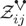is in CTC−1−κ for κ > 0 and T > 0.

Let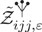be the stationary solution to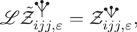, i.e.,

Set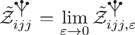where the limit is in $CTC12−κ$. To define the “next order perturbative terms,” let $D =def m−Δ$ and define

Here, c1 equals $12$ if i = kj = or i = j = k, equals 1 if i = k = j = , and is 0 otherwise, and c2 equals 1 if j = ki, equals 3 if j = k = i, and equals 0 otherwise. In addition,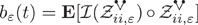and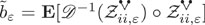are renormalization constants and $|bε−b̃ε|≲t−γ$ for any γ > 0 uniformly in ɛ. We denote collectively as

Then, we can prove that each of these objects converges in probability as ɛ → 0 in $CTCατ$, where for each tree τ, $ατ=(−52−κ)#noises+2#edges$. For example, for τ =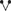, ατ = −1 − 2κ.

Before decomposing Φ, an important idea in Ref. 66 is to introduce one more auxiliary object Xi defined via the following equation:

With the stochastic objects at hand, we have the following decompositions:

$Φi=Zi+Xi+Yi,$

with Yi satisfying the following equation:94

Here, the first line in (8.7) is the expansion of $(Yj+Xj)2(Yi+Xi+Zi)$; the terms containing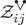and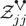correspond to the remaining terms in the paraproduct expansion of 2(Yj + Xj)ZjZi and $(Yi+Xi)Zj2$, respectively.

Recall that $D=m−Δ$, and we define

where in the last step we defined Pi. We can, then, prove the following energy identity.

It is straightforward to derive the above energy identity, at least formally. In this derivation, however, new renormalization would appear to be necessary when we take the inner product, but in the end, they cancel each other. See Ref. 92 for details.

The next step, similarly as in the 2D case, is to prove the uniformity in N estimates based on Lemma 8.1.

The main results are Theorems 8.2 and 8.3. The key step to prove these theorems is to obtain the following bound:

$∫0T(Θ+Ξ)dt⩽δ∑j=1N‖∇φj‖LT2L22+∑j=1N‖Yj‖LT2H1−2κ2+λN∑i=1NYi2LT2L22+Cδλ(1+λ55)∫0T∑i=1N‖Yi‖L22(RN1+RN2+QN3)ds+λ(1+λ)QN4$
(8.9)

for a small constant δ > 0 with certain quantities $RN1,RN2,QN3,QN4$, which only depend on the “perturbative objects” (8.5) and X in (8.6). The main technical step is, then, to show the following.

Theorem 8.2.
There exists a constantC > 0 independent ofN,λ, andm ⩾ 1 such that
$∑j=1N‖Yj(T)‖L22+12∑j=1N‖∇φj‖LT2L22+m∑j=1N‖Yj‖LT2L22+18∑j=1N‖Yj‖LT2H1−2κ2+λN∑i=1NYi2LT2L22⩽∑j=1N‖Yj(0)‖L22+λ(1+λ)QN4+∑j=1N‖Yj‖LT2L22+Cλ(1+λ55)∫0T∑i=1N‖Yi‖L22(RN1+RN2+QN3)ds.$

Furthermore, by using the dissipation effect from the term $1N‖∑i=1NYi2‖LT2L22$, the empirical averages of the L2 norms of Yi can be controlled pathwise in terms of a $Z$ dependent quantity $Q(Z)$ with finite moment, as stated in the following theorem.

Theorem 8.3.
There exists$Q(Z)$with$EQ(Z)⩽C$independent ofNsuch that
$supt∈[0,T]1N∑j=1N‖Yj(t)‖L22+mN∑j=1N‖Yj‖LT2L22+12N∑j=1N‖∇φj‖LT2L22+18N∑j=1N‖Yj‖LT2H1−2κ2+λ2N2∑i=1NYi2LT2L22⩽Q(Z)+2N∑i=1N‖Yi(0)‖L22.$

The above bounds are inputs of the proof of convergence of measures, which we discuss below.

The proof of convergence of the renormalized linear sigma model in 3D to the Gaussian free field is based on the similar idea as in the 2D case, Sec. VI. However, there are several new technical issues in 3D, which we now explain.

Recall that in 3D, we have a new decomposition

$Φi=Zi+Xi+Yi,$

where Xi solves (8.6) and Yi solves (8.7). Similarly, as in Sec. VI, we consider a stationary process $(Φi,Zi)1⩽i⩽N$ such that the components Φi are the limiting solution to (8.2) and Zi is the stationary solution to $LZi=ξi$. Similarly, as in (6.9), the convergence of measures eventually boils down to proving

$E‖Φ̃i(0)−Zi(0)‖H12−2κ2=1t∫0tE‖Φ̃i(s)−Zi(s)‖H12−2κ2ds⩽2t∫0tE‖Xi(s)‖H12−2κ2ds+2t∫0tE‖Yi(s)‖H12−2κ2ds⩽?CN−1.$
(8.10)

For the term with X, it turns out that we have

$∑i=1N‖Xi‖LT2H12−2κ2≲λ2QN0,$
(8.11)

where $QN0$ only involves the “perturbative objects,”

and we have $E|QN0|q≲1$ for every q ⩾ 1 uniformly in N. The nontriviality of (8.11) is that the left-hand side appears to be O(N), but the right-hand side is of order O(1), despite the fact that $QN0$ appears to be “three sums times 1/N2.” Thus, we essentially gain a factor of 1/N. (8.11) immediately gives

$1t∫0tE‖Xi(s)‖H12−2κ2ds=1tN∫0tE∑i=1N‖Xi(s)‖H12−2κ2ds≲1tNEQN0≲CttN,$
(8.12)

so we get the desired estimate for the first term in (8.10).

The proof of (8.11) is based on a priori estimates on the PDE (8.6). We only demonstrate why the expectation of $QN0$ is bounded uniformly in N. We have for $s=12−2κ$,

We have three summation indices and a factor 1/N2. However, if i, j1, j2 are all different by independence and the fact that Wick products are mean zero, the terms are zero. [Note that this comes from the same idea as (6.10) in the 2D case.]

We remark that the same mechanism also applies to higher order perturbative objects, such as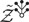(see Ref. 92, Lemma 3.4).

Next, we turn to the term with Y in (8.10). The start-point is similar to (6.11), but the analysis is more involved. From Theorem 8.2, we can deduce a bound of the form

$18∫0tE∑i=1N‖Yi(s)‖H1−2κ2ds+(m−1)∫0tE∑i=1N‖Yi(s)‖L22ds+λNE∫0t∑i=1NYi2L22ds⩽2NE‖Φ̃i(0)−Zi(0)‖L22+2∑i=1NE‖Xi(0)‖L22+Ct+Cλ∫0tE∑i=1N‖Yi(s)‖L22ERNds+Cλ∫0tE∑i=1N‖Yi(s)‖L22RN−E[RN]ds.$
(8.13)

Here, C is independent of λ, m, and N.

The quantity RN has a complicated expression, which we do not give here, but the only point that matters is that it is defined via the perturbative objects in Sec. VIII A. Moreover, all the terms in RNERN are summations of terms of the form

$1Nl∑i1⋯il=1NMi1,…,il$

for a certain choice of l, where each $Mi1,…,il$ is mean-zero and has bounded second moment, and they satisfy a crucial independence condition: $Mi1,…,il$ and $Mj1,…,jl$ are independent when the 2l indices i1, …, il, j1, …, jl are all different. For any such sum, one can prove that

$E1Nl∑i1⋯il=1NMi1,…,il2⩽C/N,$

where C only depends on l and is independent of N. From these estimates, we can eventually deduce (8.10); see Ref. 92, Sec. 5 for details.

We remark that we can also show the tightness of some observables in suitable Besov spaces in 3D; see Ref. 92, Theorem 1.2.

We complete this Review by discussing some possible future problems.

1. For the linear σ-model on a three-dimensional torus, we only studied stationary solutions and their large N limit in Ref. 92. It would be interesting to generalize the mean field limit for the dynamics in 2D discussed in Sec. V to 3D.

2. In 2D, besides the tightness of the two observables in (7.1), we also prove exact formulas for their correlations as N (Theorem 7.1); it would be interesting to prove exact correlation formulas for other O(N)-invariant observables in the large N limit. In 3D, we only obtained the tightness of observables $1N∑i=1N:Φi2:$ as random fields when N becomes large in Ref. 92, so it would be interesting to derive the exact correlation formulas for it in the large N limit.

3. It would be interesting to obtain a 1/N expansion for correlations of the field or the observables and prove bounds on the remainder of this 1/N expansion. In 2D, one might be able to incorporate the dual field approach discussed in Sec. I C as in the work of Kupiainen (Ref. 29, Sec. 4) and, then, bound the remainder of this 1/N expansion using PDE estimates. In fact, for the usual perturbation expansion for $Φ24$, we have already given new bounds on the remainder (which implies asymptoticity of the expansion) in Ref. 95.

4. Could any results discussed in this Review be generalized to infinite volume?

5. For fermionic models, could we make any prediction by physicists, e.g., Ref. 12, rigorous? Recently, the authors of Ref. 96 proposed a rigorous framework for stochastic quantization of fermionic fields (at least on lattice), which might be a reasonable start-point.

6. It would be interesting to study large N behavior of matrix-valued dynamics or develop estimates on the dynamics to deduce large N results on matrix-valued QFT (e.g., Ref. 21), for instance, (1.1) with Φ being matrix valued, either on lattice or in continuum. Besides vector and matrix models, tensor models and their large N problems were also proposed in physics.

7. Among the matrix models, Yang–Mills models are of great interest; see the recent progress on large N problems for lattice Yang–Mills model in Refs. 47 and 48. In equilibrium, Wilson loop observables converge to the deterministic limit, i.e., master fields. It would be interesting to use the dynamic to study such questions: in Ref. 97, the deterministic large N limit was shown using the dynamic, and it would be interesting to study higher order 1/N corrections. Another possible question is to obtain a certain deterministic limit for the dynamics as N. Reference 54 is also an initial attempt where the master loop equation for finite N is derived using the stochastic dynamic on lattice. On a two- and three-dimensional continuum torus, these dynamics are constructed for short time.98,99

H.S. acknowledges the financial support from the NSF under Grant Nos. DMS-1954091 and CAREER DMS-2044415 and collaborations with Scott Smith, Rongchan Zhu, and Xiangchan Zhu.

The author has no conflicts to disclose.

Hao Shen: Formal analysis (equal); Investigation (equal); Methodology (equal); Writing – original draft (equal); Writing – review & editing (equal).

Data sharing is not applicable to this article as no new data were created or analyzed in this study.

1.
H. E.
Stanley
, “
Spherical model as the limit of infinite spin dimensionality
,”
Phys. Rev.
176
(
2
),
718
(
1968
).
2.
T. H.
Berlin
and
M.
Kac
, “
The spherical model of a ferromagnet
,”
Phys. Rev.
86
(
6
),
821
(
1952
).
3.
R. J.
Baxter
,
Exactly Solved Models in Statistical Mechanics
(
Elsevier
,
2016
).
4.
M.
Kac
and
C. J.
Thompson
, “
Spherical model and the infinite spin dimensionality limit
,”
Phys. Norv.
5
(
3–4
),
163
168
(
1971
).
5.
P. A.
Pearce
and
C. J.
Thompson
, “
The spherical limit for n-vector correlations
,”
J. Stat. Phys.
17
(
4
),
189
196
(
1977
).
6.
M. V.
Shcherbina
, “
The spherical limit of n-vector correlations
,”
Theor. Math.
77
(
3
),
1323
1331
(
1988
).
7.
E.
Brézin
and
D. J.
Wallace
, “
Critical behavior of a classical Heisenberg ferromagnet with many degrees of freedom
,”
Phys. Rev. B
7
(
5
),
1967
(
1973
).
8.
K. G.
Wilson
, “
Quantum field-theory models in less than 4 dimensions
,”
Phys. Rev. D
7
(
10
),
2911
(
1973
).
9.
S.
Coleman
,
R.
Jackiw
, and
H. D.
Politzer
, “
Spontaneous symmetry breaking in the O(N) model for large N
,”
Phys. Rev. D
10
(
8
),
2491
(
1974
).
10.
L. F.
Abbott
,
J. S.
Kang
, and
H. J.
Schnitzer
, “
Bound states, tachyons, and restoration of symmetry in the 1/N expansion
,”
Phys. Rev. D
13
(
8
),
2212
(
1976
).
11.

Remark that in this Review, we will not discuss phase transition or spontaneous symmetry breaking although it is an interesting topic.

12.
D. J.
Gross
and
A.
Neveu
, “
Dynamical symmetry breaking in asymptotically free field theories
,”
Phys. Rev. D
10
(
10
),
3235
(
1974
).
13.
W. A.
Bardeen
,
B. W.
Lee
, and
R. E.
Shrock
, “
Phase transition in the nonlinear σ model in a (2 + ɛ)-dimensional continuum
,”
Phys. Rev. D
14
(
4
),
985
(
1976
).
14.
A.
,
P.
Di Vecchia
, and
M.
Lüscher
, “
Confinement and chiral symmetry breaking in CPn−1 models with quarks
,”
Nucl. Phys. B
152
(
1
),
125
144
(
1979
).
15.
A.
,
M.
Lüscher
, and
P.
Di Vecchia
, “
A 1/n expandable series of non-linear σ models with instantons
,”
Nucl. Phys. B
146
(
1
),
63
76
(
1978
).
16.
G.
t’Hooft
, “
A planar diagram theory for strong interactions
,”
Nucl. Phys. B
72
(
3
),
461
473
(
1974
).
17.
V. A.
Kazakov
, “
Wilson loop average for an arbitrary contour in two-dimensional U(N) gauge theory
,”
Nucl. Phys. B
179
(
2
),
283
292
(
1981
).
18.
V. A.
Kazakov
and
I. K.
Kostov
, “
Non-linear strings in two-dimensional U(∞) gauge theory
,”
Nucl. Phys. B
176
(
1
),
199
215
(
1980
).
19.
Y. M.
Makeenko
and
A. A.
Migdal
, “
Exact equation for the loop average in multicolor QCD
,”
Phys. Lett. B
88
(
1–2
),
135
137
(
1979
).
20.
A. M.
Polyakov
, “
Gauge fields as rings of glue
,”
Nucl. Phys. B
164
,
171
188
(
1980
).
21.
R.
Gopakumar
and
D. J.
Gross
, “
Mastering the master field
,”
Nucl. Phys. B
451
(
1–2
),
379
415
(
1995
).
22.
E.
Brezin
and
S. R.
,
The Large N Expansion in Quantum Field Theory and Statistical Physics: From Spin Systems to 2-dimensional Gravity
(
World Scientific
,
1993
).
23.
E.
Witten
, “
The 1/N expansion in atomic and particle physics
,” in
Recent Developments in Gauge Theories
(
Springer
,
1980
), pp.
403
419
.
24.
M.
Moshe
and
J.
Zinn-Justin
, “
Quantum field theory in the large N limit: A review
,”
Phys. Rep.
385
(
3–6
),
69
228
(
2003
).
25.
S.
Coleman
,
Aspects of Symmetry: Selected Erice Lectures
(
Cambridge University Press
,
1988
).
26.
M. E.
Peskin
and
D. V.
Schroeder
,
An Introduction to Quantum Field Theory
(
,
,
1995
), Edited and with a foreword by David Pines.
27.

We will have more precise discussion on Wick renormalization in Sec. IV.

28.
K.
Symanzik
, “
1/N expansion in $P(φ2)4−ϵ$ theory I. Massless theory 0 < ϵ < 2
,”
Deutsches Elektronen-Synchrotron (DESY)
77
(
05
) (
1977
).
29.
A. J.
Kupiainen
, “
1/n expansion for a quantum field model
,”
Commun. Math. Phys.
74
(
3
),
199
222
(
1980
).
30.
A.
Guionnet
,
Asymptotics of Random Matrices and Related Models: The Uses of Dyson-Schwinger Equations
(
American Mathematical Society
,
2019
), Vol. 130.
31.
A. J.
Kupiainen
, “
On the 1/n expansion
,”
Commun. Math. Phys.
73
(
3
),
273
294
(
1980
).
32.
A.
Kupiainen
, “
1/n expansion—some rigorous results
,” in
Mathematical Problems in Theoretical Physics (Proceedings of the International Conference on Mathematical Physics, Lausanne, 1979)
, Lecture Notes in Physics Vol. 116 (
Springer
,
Berlin; New York
,
1980
), pp.
208
210
.
33.
J.
Fröhlich
and
B.
Simon
, “
Pure states for general P(ϕ)2 theories: Construction, regularity and variational equality
,”
Ann. Math.
105
(
3
),
493
526
(
1977
).
34.
C.
Billionnet
and
P.
Renouard
, “
Analytic interpolation and Borel summability of the $(λNΦN:4)2$ models. I. Finite volume approximation
,”
Commun. Math. Phys.
84
(
2
),
257
295
(
1982
).
35.
D.
Brydges
and
P.
Federbush
, “
A lower bound for the mass of a random Gaussian lattice
,”
Commun. Math. Phys.
62
(
1
),
79
82
(
1978
).
36.
J.
Fröhlich
,
A.
Mardin
, and
V.
Rivasseau
, “
Borel summability of the 1/N expansion for the N-vector [O(N) nonlinear σ] models
,”
Commun. Math. Phys.
86
(
1
),
87
110
(
1982
).
37.
C.
Kopper
,
J.
Magnen
, and
V.
Rivasseau
, “
Mass generation in the large N Gross-Neveu-model
,”
Commun. Math. Phys.
169
(
1
),
121
180
(
1995
).
38.
K. R.
Ito
and
H.
Tamura
, “
N dependence of upper bounds of critical temperatures of 2D O(N) spin models
,”
Commun. Math. Phys.
202
(
1
),
127
168
(
1999
).
39.
C.
Kopper
, “
Mass generation in the large N-nonlinear σ-model
,”
Commun. Math. Phys.
202
(
1
),
89
126
(
1999
).
40.
T.
Lévy
, “
The master field on the plane
,”
Astérisque
388
,
ix+201
(
2017
).
41.
I. M.
Singer
, “
On the master field in two dimensions
,” in
Functional Analysis on the Eve of the 21st Century: Vol. 1 (New Brunswick, NJ, 1993)
, Progress in Mathematics Vol. 131 (
Birkhäuser
,
Boston, MA
,
1995
), pp.
263
281
.
42.
T.
Lévy
, “
Yang-Mills measure on compact surfaces
,”
Mem. Am. Math. Soc.
166
,
790
(
2003
).
43.
T.
Lévy
, “
Two-dimensional Markovian holonomy fields
,”
Astérisque
329
,
172
(
2010
).
44.
M.
Anshelevich
and
A. N.
Sengupta
, “
Quantum free Yang–Mills on the plane
,”
J. Geom. Phys.
62
(
2
),
330
343
(
2012
).
45.
A.
Sengupta
,
Gauge Theory on Compact Surfaces
, Memoirs of the American Mathematical Society (
1997
), Vol. 126, Issue 600, pp.
viii+85
.
46.
A. N.
Sengupta
, “
The large-N Yang-Mills field on the plane and free noise
,”
AIP Conf. Proc.
1079
,
121
132
(
2008
).
47.
S.
Chatterjee
, “
Rigorous solution of strongly coupled SO(N) lattice gauge theory in the large N limit
,”
Commun. Math. Phys.
366
(
1
),
203
268
(
2019
).
48.
S.
Chatterjee
and
J.
Jafarov
, “
The 1/N expansion for SO(N) lattice gauge theory at strong coupling
,” arXiv:1604.04777 (
2016
).
49.
A.
Dahlqvist
, “
Free energies and fluctuations for the unitary Brownian motion
,”
Commun. Math. Phys.
348
(
2
),
395
444
(
2016
).
50.
B. K.
Driver
, “
A functional integral approaches to the Makeenko-Migdal equations
,”
Commun. Math. Phys.
370
(
1
),
49
116
(
2019
).
51.
B. K.
Driver
,
F.
Gabriel
,
B. C.
Hall
, and
T.
Kemp
, “
The Makeenko–Migdal equation for Yang-Mills theory on compact surfaces
,”
Commun. Math. Phys.
352
(
3
),
967
978
(
2017
).
52.
B. K.
Driver
,
B. C.
Hall
, and
T.
Kemp
, “
Three proofs of the Makeenko–Migdal equation for Yang–Mills theory on the plane
,”
Commun. Math. Phys.
351
(
2
),
741
774
(
2017
).
53.
J.
Jafarov
, “
Wilson loop expectations in SU(N) lattice gauge theory
,” arXiv:1610.03821 (
2016
).
54.
H.
Shen
,
S. A.
Smith
, and
R.
Zhu
, “
A new derivation of the finite N master loop equation for lattice Yang–Mills
,” arXiv:2202.00880 (
2022
).
55.
G.
Parisi
and
Y. S.
Wu
, “
Perturbation theory without gauge fixing
,”
Sci. Sintering
24
(
4
),
483
496
(
1981
).
56.

One even simpler example in stochastic ordinary differential equations is given by the Ornstein–Uhlenbeck process $dXt=−12Xtdt+dBt$, where Bt is the Brownian motion, and its invariant measure is the (one-dimensional) Gaussian measure $12πe−X22dX$.

57.
J.
Glimm
and
A.
Jaffe
,
Quantum Physics: A Functional Integral Point of View
, 2nd ed. (
Springer-Verlag
,
New York
,
1987
).
58.
A.
Jaffe
, “
Constructive quantum field theory
,”
Math. Phys.
2000
,
111
127
.
59.
S.
Albeverio
and
M.
Röckner
, “
Stochastic differential equations in infinite dimensions: Solutions via Dirichlet forms
,”
Probab. Theory Relat. Fields
89
(
3
),
347
386
(
1991
).
60.
G.
Da Prato
and
A.
Debussche
, “
Strong solutions to the stochastic quantization equations
,”
Ann. Probab.
31
(
4
),
1900
1916
(
2003
).
61.
J.-C.
Mourrat
and
H.
Weber
, “
Global well-posedness of the dynamic Φ4 model in the plane
,”
Ann. Probab.
45
(
4
),
2398
2476
(
2017
).
62.
M.
Hairer
, “
A theory of regularity structures
,”
Inventiones Math.
198
(
2
),
269
504
(
2014
).
63.
R.
Catellier
and
K.
Chouk
, “
Paracontrolled distributions and the 3-dimensional stochastic quantization equation
,”
Ann. Probab.
46
(
5
),
2621
2679
(
2018
).
64.
J.-C.
Mourrat
and
H.
Weber
, “
The dynamic $Φ34$ model comes down from infinity
,”
Commun. Math. Phys.
356
(
3
),
673
753
(
2017
).
65.
M.
Gubinelli
and
M.
Hofmanová
, “
Global solutions to elliptic and parabolic Φ4 models in Euclidean space
,”
Commun. Math. Phys.
368
(
3
),
1201
1266
(
2019
).
66.
M.
Gubinelli
and
M.
Hofmanová
, “
A PDE construction of the Euclidean $ϕ34$ quantum field theory
,”
Commun. Math. Phys.
384
(
1
),
1
75
(
2021
).
67.
S.
Albeverio
and
S.
Kusuoka
, “
The invariant measure and the flow associated to the $ϕ34$-quantum field model
,”
Ann. Sc. Norm. Super. Pisa-Cl. Sci.
20
(
4
),
1359
1427
(
2020
).
68.
A.
Moinat
and
H.
Weber
, “
Space-time localisation for the dynamic $ϕ34$ model
,”
Commun. Pure Appl. Math.
73
(
12
),
2519
2555
(
2020
).
69.
J.
Alfaro
, “
Stochastic quantization and the large-N reduction of U(N) gauge theory
,”
Phys. Rev. D
28
(
4
),
1001
(
1983
).
70.
J.
Alfaro
and
B.
Sakita
, “
Derivation of quenched momentum prescription by means of stochastic quantization
,”
Phys. Lett. B
121
(
5
),
339
344
(
1983
).
71.
P. H.
Damgaard
and
H.
Hüffel
, “
Stochastic quantization
,”
Phys. Rep.
152
(
5–6
),
227
398
(
1987
).
72.
H. P.
McKean
, “
Propagation of chaos for a class of non-linear parabolic equations
,” in
Stochastic Differential Equations
, Lecture Series in Differential Equations, Session 7, Catholic University, 1967 (
Air Force Office of Scientific Research
,
Arlington, VA
,
1967
), pp.
41
57
.
73.
A.-S.
Sznitman
, “
Topics in propagation of chaos
,” in
École d’Été de Probabilités de Saint-Flour XIX—1989
, Lecture Notes in Mathematics Vol. 1464 (
Springer
,
Berlin
,
1991
), pp.
165
251
.
74.

In the context of SDE systems, one also considers the empirical measures of the particle configurations and aims to show their convergence as N to the McKean–Vlasov PDEs, which are typically deterministic. Note that in this Review, we do not consider the “analog” of McKean–Vlasov PDE (which would be infinite dimensional) in the context of our model.

75.
I.
Bailleul
,
R.
Catellier
, and
F.
Delarue
, “
Solving mean field rough differential equations
,”
Electron. J. Probab.
25
,
1
51
(
2020
).
76.
T.
Cass
and
T.
Lyons
, “
Evolving communities with individual preferences
,”
Proc. London Math. Soc.
110
(
1
),
83
107
(
2015
).
77.
M.
Coghi
,
J.-D.
Deuschel
,
P. K.
Friz
, and
M.
Maurelli
, “
Pathwise McKean–Vlasov theory with additive noise
,”
Ann. Appl. Probab.
30
(
5
),
2355
2392
(
2020
).
78.
J.-M.
Lasry
and
P.-L.
Lions
, “
Mean field games
,”
Jpn. J. Math.
2
(
1
),
229
260
(
2007
).
79.
L.
Erdős
,
B.
Schlein
, and
H.-T.
Yau
, “
Derivation of the Gross-Pitaevskii equation for the dynamics of Bose-Einstein condensate
,”
Ann. Math.
172
(
1
),
291
370
(
2010
).
80.
F.
Golse
, “
On the dynamics of large particle systems in the mean field limit
,” in
Macroscopic and Large Scale Phenomena: Coarse Graining, Mean Field Limits and Ergodicity
, Lecture Notes in Applied Mathematics and Mechanics Vol. 3 (
Springer
,
Cham
,
2016
), pp.
1
144
.
81.
P.-E.
Jabin
, “
A review of the mean field limits for Vlasov equations
,”
Kinet. Relat. Models
7
(
4
),
661
711
(
2014
).
82.
H.
Spohn
,
Large Scale Dynamics of Interacting Particles
, Texts and Monographs in Physics (
Springer
,
1991
).
83.
G.
Kallianpur
and
J.
Xiong
,
Stochastic Differential Equations in Infinite-Dimensional Spaces
, Institute of Mathematical Statistics Lecture Notes—Monograph Series Vol. 26 (
Institute of Mathematical Statistics
,
Hayward, CA
,
1995
).
84.
W.
E
and
H.
Shen
, “
Mean field limit of a dynamical model of polymer systems
,”
Sci. China Math.
56
(
12
),
2591
2598
(
2013
).
85.

The renormalization constant here for our SPDE is consistent with the one in QFT, which is well known in physics.

86.

We will assume sufficiently regular initial condition ϕ in this section and focus on roughness of the equation itself for simplicity.

87.

Here, we denote by Cα the Hölder–Besov spaces; see, e.g., Ref. 90, Appendix A for the definitions of these spaces.

88.
H.
Bahouri
,
J.-Y.
Chemin
, and
R.
Danchin
.
Fourier Analysis and Nonlinear Partial Differential Equations
, Grundlehren der Mathematischen Wissenschaften [Fundamental Principles of Mathematical Sciences] Vol. 343 (
Springer
,
Heidelberg
,
2011
).
89.

Recall that this means that their joint probability distribution does not change under permutations of the components.

90.
H.
Shen
,
S. A.
Smith
,
R.
Zhu
, and
X.
Zhu
, “
Large N limit of the O(N) linear sigma model via stochastic quantization
,”
Ann. Probab.
50
(
1
),
131
202
(
2022
).
91.

The block Δjf is basically the Fourier modes of order 2j of f.

92.
H.
Shen
,
R.
Zhu
, and
X.
Zhu
, “
Large N limit of the O(N) linear sigma model in 3D
,”
Commun. Math. Phys.
394
,
953
1009
(
2022
).
93.

These renormalization constants are the same as in the one-component $Φ34$ QFT, namely, aɛ diverges at rate ɛ−1 and $b̃ε$ diverges logarithmically.

94.

In fact, some products in (8.7) are understood via renormalization; see Ref. 92 for details.

95.
H.
Shen
,
R.
Zhu
, and
X.
Zhu
, “
An SPDE approach to perturbation theory of $Φ24$: Asymptoticity and short distance behavior
,” arXiv:2108.11312 (
2021
).
96.
S.
Albeverio
,
L.
Borasi
,
F. C.
De Vecchi
, and
M.
Gubinelli
, “
Grassmannian stochastic analysis and the stochastic quantization of Euclidean fermions
,”
Probab. Theory Relat. Fields
183
(
3--4
),
909
995
(
2022
).
97.
H.
Shen
,
R.
Zhu
, and
X.
Zhu
, “
A stochastic analysis approach to lattice Yang–Mills at strong coupling
,” arXiv:2204.12737 (
2022
).
98.
A.
Chandra
,
I.
Chevyrev
,
M.
Hairer
, and
H.
Shen
, “
Langevin dynamic for the 2D Yang-Mills measure
,”
Publ. Math. IHES
(published online).
99.
A.
Chandra
,
I.
Chevyrev
,
M.
Hairer
, and
H.
Shen
,”
Stochastic quantisation of Yang-Mills-Higgs in 3D
,” arXiv:2201.03487 (
2022
).